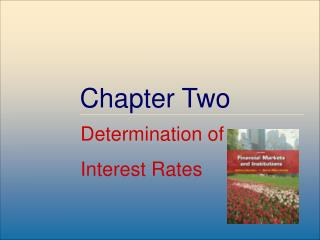DownloadDownload PresentationChapter Two

# Chapter Two

Télécharger la présentation## Chapter Two

- - - - - - - - - - - - - - - - - - - - - - - - - - - E N D - - - - - - - - - - - - - - - - - - - - - - - - - - -
##### Presentation Transcript

1. Chapter Two Determination of Interest Rates McGraw-Hill/Irwin

2. Interest Rate Fundamentals • Nominal interest rates: the interest rates actually observed in financial markets • affect the values (prices) of securities traded in money and capital markets • affect the relationships between spot and forward FX rates McGraw-Hill/Irwin

3. Time Value of Money and Interest Rates • The time value of money is based on the notion that a dollar received today is worth more than a dollar received at some future date • Simple interest: interest earned on an investment is not reinvested • Compound interest: interest earned on an investment is reinvested McGraw-Hill/Irwin

4. Present Value of a Lump Sum • Discount future payments using current interest rates to find the present value (PV) PV = FVt[1/(1 + r)]t = FVt(PVIFr,t) PV = present value of cash flow FVt = future value of cash flow (lump sum) received in t periods r= interest rate per period t= number of years in investment horizon PVIFr,t = present value interest factor of a lump sum McGraw-Hill/Irwin

5. Future Value of a Lump Sum • The future value (FV) of a lump sum received at the beginning of an investment horizon FVt = PV(1 + r)t = PV(FVIFr,t) FVIFr,t = future value interest factor of a lump sum McGraw-Hill/Irwin

6. Relation between Interest Rates and Present and Future Values Present Value (PV) Future Value (FV) Interest Rate Interest Rate McGraw-Hill/Irwin

7. Present Value of an Annuity • The present value of a finite series of equal cash flows received on the last day of equal intervals throughout the investment horizon PMT = periodic annuity payment PVIFAr,t = present value interest factor of an annuity McGraw-Hill/Irwin

8. Future Value of an Annuity • The future value of a finite series of equal cash flows received on the last day of equal intervals throughout the investment horizon FVIFAr,t = future value interest factor of an annuity McGraw-Hill/Irwin

9. Effective Annual Return • Effective or equivalent annual return (EAR) is the return earned or paid over a 12-month period taking compounding into account EAR= (1 + r)c – 1 c = the number of compounding periods per year McGraw-Hill/Irwin

10. Financial Calculators • Setting up a financial calculator • Number of digits shown after decimal point • Number of compounding periods per year • Key inputs/outputs (solve for one of five) N = number of compounding periods I/Y = annual interest rate PV = present value (i.e., current price) PMT = a constant payment every period FV = future value (i.e., future price) McGraw-Hill/Irwin

11. Loanable Funds Theory • Loanable funds theory explains interest rates and interest rate movements • Views level of interest rates in financial markets as a result of the supply and demand for loanable funds • Domestic and foreign households, businesses, and governments all supply and demand loanable funds McGraw-Hill/Irwin

12. Supply and Demand of Loanable Funds Demand Supply Interest Rate Quantity of Loanable Funds Supplied and Demanded McGraw-Hill/Irwin

13. Shifts in Supply and Demand Curves change Equilibrium Interest Rates Increased supply of loanable funds Increased demand for loanable funds Interest Rate DD* Interest Rate SS SS DD DD SS* i** E* E i* E i* E* i** Q* Q** Q* Q** Quantity of Funds Supplied Quantity of Funds Demanded McGraw-Hill/Irwin

14. Determinants of Interest Rates for Individual Securities • ij* = f(IP, RIR, DRPj, LRPj, SCPj, MPj) • Inflation (IP) IP = [(CPIt+1) – (CPIt)]/(CPIt) x (100/1) • Real Interest Rate (RIR) and the Fisher effect RIR = i – Expected (IP) McGraw-Hill/Irwin

15. Determinants of Interest Rates for Individual Securities (cont’d) • Default Risk Premium (DRP) DRPj = ijt – iTt ijt = interest rate on securityj at time t iTt = interest rate on similar maturity U.S. Treasury security at time t • Liquidity Risk (LRP) • Special Provisions (SCP) • Term to Maturity (MP) McGraw-Hill/Irwin

16. Term Structure of Interest Rates:the Yield Curve (a) Upward sloping (b) Inverted or downward sloping (c) Flat Yield to Maturity (a) (c) (b) Time to Maturity McGraw-Hill/Irwin

17. Unbiased Expectations Theory • Long-term interest rates are geometric averages of current and expected future short-term interest rates 1RN= actual N-period rate today N= term to maturity, N = 1, 2, …, 4, … 1R1= actual current one-year rate today E(ir1)= expected one-year rates for years, i = 1 to N McGraw-Hill/Irwin

18. Liquidity Premium Theory • Long-term interest rates are geometric averages of current and expected future short-term interest rates plus liquidity risk premiums that increase with maturity Lt= liquidity premium for periodt L2 < L3 < …<LN McGraw-Hill/Irwin

19. Market Segmentation Theory • Individual investors and FIs have specific maturity preferences • Interest rates are determined by distinct supply and demand conditions within many maturity segments • Investors and borrowers deviate from their preferred maturity segment only when adequately compensated to do so McGraw-Hill/Irwin

20. Implied Forward Rates • A forward rate (f) is an expected rate on a short-term security that is to be originated at some point in the future • The one-year forward rate for any year N in the future is: McGraw-Hill/Irwin